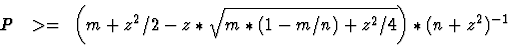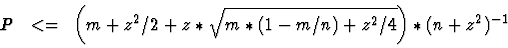## Appendix B  --  Bounds on the Best Change'' Probabilities

The Best Change'' behaviours of chess programs represent typical count data for a binary-valued random variable in the terms of standard statistics. The count probabilities of binary-valued random variables generally adhere to binomial distributions. For large enough sample sizes n and success counts m with m > 4 and n - m > 4, however, corresponding normal distributions provide practically sufficient approximations of the awkward to handle binomial distributions. Classic engineering statistics  derive the following lower and upper bounds of the success probability P for given values of m, n and any desired %-level of confidence as specified by the single-sided percentiles z of the N(0, 1) normal distribution.(1.1)(1.2)

With the help of these formulas we determined 80%-confident (z = 0.8416) and 90%-confident (z = 1.2816) bounds on the Best Change'' probabilities of BELLE (n = 447), CRAFTY (n = 343), and DARKTHOUGHT (n = 343). For BELLE we calculated the success count m from its Best Change'' rates of Table 1.4. For CRAFTY and DARKTHOUGHT we used the absolute Best Change'' numbers of Table 1.5 and Table 1.6 as their observed success counts m. The resulting bounds clearly discriminate the drops of the Best Change'' rates below 20% for all three programs with at least 80% confidence (see Table 1.13 where  >= denotes lower bounds and  <= upper bounds).

Table 1.13: Confident Bounds on the Best Change'' Probabilities.
 Search Belle Belle Crafty Crafty DarkTh. DarkTh. Depth C=80% C=90% C=80% C=90% C=80% C=90% 2 - - - - >= 36.59% >= 35.46% >= 33.14% >= 32.05% 3 - - - - >= 34.57% >= 33.47% >= 37.45% >= 36.32% 4 >= 31.26% >= 30.32% >= 28.56% >= 27.52% >= 29.70% >= 28.65% 5 >= 31.26% >= 30.32% >= 28.27% >= 27.24% >= 27.42% >= 26.40% 6 >= 25.99% >= 25.11% >= 25.43% >= 24.43% >= 22.59% >= 21.64% 7 >= 27.75% >= 26.84% >= 22.59% >= 21.64% >= 19.48% >= 18.59% 8 >= 24.24% >= 23.38% >= 20.61% >= 19.70% >= 23.16% >= 22.20% 9 >= 20.97% >= 20.16% <= 20.19% <= 21.20% >= 19.76% >= 18.87% 10 <= 19.24% <= 20.10% <= 18.98% <= 19.97% >= 22.31% >= 21.36% 11 <= 19.70% <= 20.57% <= 18.38% <= 19.35% <= 19.29% <= 20.27% 12 - - - - <= 18.68% <= 19.66% <= 17.17% <= 18.12% 13 - - - - <= 16.25% <= 17.19% <= 18.38% <= 19.35% 14 - - - - <= 17.17% <= 18.12% <= 15.34% <= 16.26%

Created by Ernst A. Heinz, Thu Dec 16 23:28:11 EST 1999